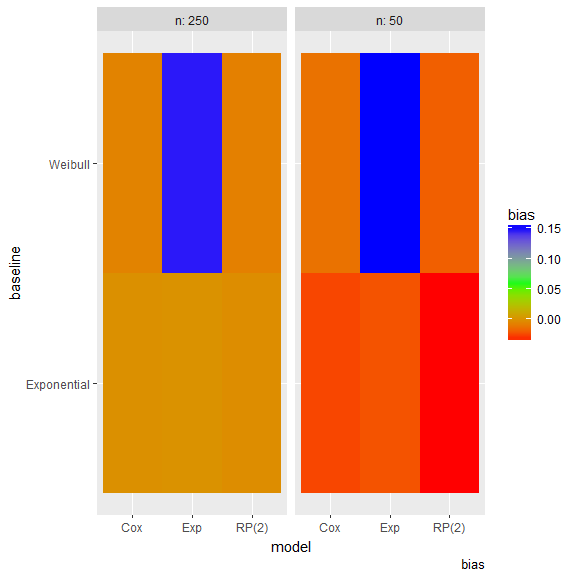# Visualising results from rsimsum

#### 2018-04-04

This vignette requires the following R packages:

library(rsimsum)
library(ggplot2)

# Simulation study with a single estimand

We use data from a simulation study on misspecification of the baseline hazard in survival models. This dataset is included in rsimsum and can be loaded with:

data("relhaz", package = "rsimsum")

Inspecting the structure of the dataset and the first 15 rows of data:

str(relhaz)
#> 'data.frame':    12000 obs. of  6 variables:
#>  $dataset : int 1 2 3 4 5 6 7 8 9 10 ... #>$ n       : num  50 50 50 50 50 50 50 50 50 50 ...
#>  $baseline: chr "Exponential" "Exponential" "Exponential" "Exponential" ... #>$ theta   : num  -0.88 -0.815 -0.143 -0.333 -0.483 ...
#>  $se : num 0.333 0.325 0.305 0.314 0.306 ... #>$ model   : chr  "Cox" "Cox" "Cox" "Cox" ...
#>    dataset  n    baseline       theta        se model
#> 1        1 50 Exponential -0.88006151 0.3330172   Cox
#> 2        2 50 Exponential -0.81460242 0.3253010   Cox
#> 3        3 50 Exponential -0.14262887 0.3050516   Cox
#> 4        4 50 Exponential -0.33251820 0.3144033   Cox
#> 5        5 50 Exponential -0.48269940 0.3064726   Cox
#> 6        6 50 Exponential -0.03160756 0.3097203   Cox
#> 7        7 50 Exponential -0.23578090 0.3121350   Cox
#> 8        8 50 Exponential -0.05046332 0.3136058   Cox
#> 9        9 50 Exponential -0.22378715 0.3066037   Cox
#> 10      10 50 Exponential -0.45326446 0.3330173   Cox
#> 11      11 50 Exponential -0.71402510 0.3251902   Cox
#> 12      12 50 Exponential -0.32956944 0.3073481   Cox
#> 13      13 50 Exponential -0.15351788 0.3056453   Cox
#> 14      14 50 Exponential -0.82742207 0.3283561   Cox
#> 15      15 50 Exponential -0.14594648 0.3255636   Cox

## Summarise results

We use the simsum function to summarise results:

s1 <- simsum(data = relhaz, estvarname = "theta", se = "se", true = -0.50, methodvar = "model", by = c("n", "baseline"), x = TRUE)
#> ref was not specified, Cox set as the reference
s1
#>
#> Call:
#>  simsum(data = relhaz, estvarname = "theta", true = -0.5, se = "se",
#>     methodvar = "model", by = c("n", "baseline"), x = TRUE)
#>
#> Method variable: model
#>  Unique methods: Cox, Exp, RP(2)
#>  Reference method: Cox
#>
#> By factors: n, baseline
#>
#> Monte Carlo standard errors were computed.

We call simsum with x = TRUE as we want to produce pattern plots and zip plots later on.

summary(s1)
#>
#> Call:
#>  simsum(data = relhaz, estvarname = "theta", true = -0.5, se = "se",
#>     methodvar = "model", by = c("n", "baseline"), x = TRUE)
#>
#> Method variable: model
#>  Unique methods: Cox, Exp, RP(2)
#>  Reference method: Cox
#> By factors: n, baseline
#>
#> Summary statistics:
#>
#>  Method = Cox, n = 250, baseline = Exponential
#>                                              Estimate   MCSE Lower 95% Upper 95%
#>  Simulations with non-missing estimates/SEs 1000.0000     NA        NA        NA
#>                      Average point estimate   -0.5004     NA        NA        NA
#>                       Median point estimate   -0.4969     NA        NA        NA
#>                      Average standard error    0.0194     NA        NA        NA
#>                       Median standard error    0.0194     NA        NA        NA
#>                      Bias in point estimate   -0.0004 0.0043   -0.0088    0.0081
#>                    Empirical standard error    0.1363 0.0030    0.1303    0.1422
#>                          Mean squared error    0.0185 0.0009    0.0168    0.0203
#>  % gain in precision relative to method Cox    1.0000 0.0000    1.0000    1.0000
#>                  Model-based standard error    0.1394 0.0001    0.1393    0.1396
#>          Relative % error in standard error    2.3293 2.2894   -2.1578    6.8164
#>                  Coverage of nominal 95% CI    0.9510 0.0068    0.9376    0.9644
#>   Bias corrected coverage of nominal 95% CI    0.9520 0.0068    0.9388    0.9652
#>                      Power of 5% level test    0.9570 0.0064    0.9444    0.9696
#>
#>  Method = Exp, n = 250, baseline = Exponential
#>                                              Estimate   MCSE Lower 95% Upper 95%
#>  Simulations with non-missing estimates/SEs 1000.0000     NA        NA        NA
#>                      Average point estimate   -0.4996     NA        NA        NA
#>                       Median point estimate   -0.5004     NA        NA        NA
#>                      Average standard error    0.0191     NA        NA        NA
#>                       Median standard error    0.0190     NA        NA        NA
#>                      Bias in point estimate    0.0004 0.0043   -0.0080    0.0088
#>                    Empirical standard error    0.1350 0.0030    0.1291    0.1409
#>                          Mean squared error    0.0182 0.0009    0.0165    0.0199
#>  % gain in precision relative to method Cox    1.0192 0.0093    1.0009    1.0375
#>                  Model-based standard error    0.1381 0.0001    0.1379    0.1382
#>          Relative % error in standard error    2.2813 2.2882   -2.2035    6.7661
#>                  Coverage of nominal 95% CI    0.9540 0.0066    0.9410    0.9670
#>   Bias corrected coverage of nominal 95% CI    0.9540 0.0066    0.9410    0.9670
#>                      Power of 5% level test    0.9570 0.0064    0.9444    0.9696
#>
#>  Method = RP(2), n = 250, baseline = Exponential
#>                                              Estimate   MCSE Lower 95% Upper 95%
#>  Simulations with non-missing estimates/SEs 1000.0000     NA        NA        NA
#>                      Average point estimate   -0.5015     NA        NA        NA
#>                       Median point estimate   -0.5000     NA        NA        NA
#>                      Average standard error    0.0194     NA        NA        NA
#>                       Median standard error    0.0193     NA        NA        NA
#>                      Bias in point estimate   -0.0015 0.0043   -0.0100    0.0069
#>                    Empirical standard error    0.1363 0.0030    0.1303    0.1423
#>                          Mean squared error    0.0186 0.0009    0.0168    0.0203
#>  % gain in precision relative to method Cox    0.9996 0.0034    0.9929    1.0063
#>                  Model-based standard error    0.1392 0.0001    0.1391    0.1394
#>          Relative % error in standard error    2.1570 2.2855   -2.3225    6.6365
#>                  Coverage of nominal 95% CI    0.9520 0.0068    0.9388    0.9652
#>   Bias corrected coverage of nominal 95% CI    0.9520 0.0068    0.9388    0.9652
#>                      Power of 5% level test    0.9590 0.0063    0.9467    0.9713
#>
#>  Method = Cox, n = 50, baseline = Exponential
#>                                              Estimate   MCSE Lower 95% Upper 95%
#>  Simulations with non-missing estimates/SEs 1000.0000     NA        NA        NA
#>                      Average point estimate   -0.5255     NA        NA        NA
#>                       Median point estimate   -0.5226     NA        NA        NA
#>                      Average standard error    0.1016     NA        NA        NA
#>                       Median standard error    0.1003     NA        NA        NA
#>                      Bias in point estimate   -0.0255 0.0104   -0.0458   -0.0052
#>                    Empirical standard error    0.3277 0.0073    0.3133    0.3421
#>                          Mean squared error    0.1079 0.0047    0.0988    0.1170
#>  % gain in precision relative to method Cox    1.0000 0.0000    1.0000    1.0000
#>                  Model-based standard error    0.3188 0.0004    0.3180    0.3197
#>          Relative % error in standard error   -2.7072 2.1801   -6.9802    1.5658
#>                  Coverage of nominal 95% CI    0.9410 0.0075    0.9264    0.9556
#>   Bias corrected coverage of nominal 95% CI    0.9400 0.0075    0.9253    0.9547
#>                      Power of 5% level test    0.3740 0.0153    0.3440    0.4040
#>
#>  Method = Exp, n = 50, baseline = Exponential
#>                                              Estimate   MCSE Lower 95% Upper 95%
#>  Simulations with non-missing estimates/SEs 1000.0000     NA        NA        NA
#>                      Average point estimate   -0.5223     NA        NA        NA
#>                       Median point estimate   -0.5181     NA        NA        NA
#>                      Average standard error    0.0977     NA        NA        NA
#>                       Median standard error    0.0970     NA        NA        NA
#>                      Bias in point estimate   -0.0223 0.0101   -0.0422   -0.0024
#>                    Empirical standard error    0.3208 0.0072    0.3068    0.3349
#>                          Mean squared error    0.1033 0.0045    0.0945    0.1122
#>  % gain in precision relative to method Cox    1.0431 0.0126    1.0183    1.0679
#>                  Model-based standard error    0.3126 0.0004    0.3118    0.3133
#>          Relative % error in standard error   -2.5836 2.1821   -6.8603    1.6932
#>                  Coverage of nominal 95% CI    0.9370 0.0077    0.9219    0.9521
#>   Bias corrected coverage of nominal 95% CI    0.9390 0.0076    0.9242    0.9538
#>                      Power of 5% level test    0.3850 0.0154    0.3548    0.4152
#>
#>  Method = RP(2), n = 50, baseline = Exponential
#>                                              Estimate   MCSE Lower 95% Upper 95%
#>  Simulations with non-missing estimates/SEs 1000.0000     NA        NA        NA
#>                      Average point estimate   -0.5337     NA        NA        NA
#>                       Median point estimate   -0.5269     NA        NA        NA
#>                      Average standard error    0.1005     NA        NA        NA
#>                       Median standard error    0.0993     NA        NA        NA
#>                      Bias in point estimate   -0.0337 0.0105   -0.0543   -0.0131
#>                    Empirical standard error    0.3323 0.0074    0.3177    0.3468
#>                          Mean squared error    0.1114 0.0049    0.1018    0.1210
#>  % gain in precision relative to method Cox    0.9726 0.0066    0.9597    0.9855
#>                  Model-based standard error    0.3171 0.0004    0.3162    0.3179
#>          Relative % error in standard error   -4.5711 2.1383   -8.7620   -0.3802
#>                  Coverage of nominal 95% CI    0.9370 0.0077    0.9219    0.9521
#>   Bias corrected coverage of nominal 95% CI    0.9390 0.0076    0.9242    0.9538
#>                      Power of 5% level test    0.3920 0.0154    0.3617    0.4223
#>
#>  Method = Cox, n = 250, baseline = Weibull
#>                                              Estimate   MCSE Lower 95% Upper 95%
#>  Simulations with non-missing estimates/SEs 1000.0000     NA        NA        NA
#>                      Average point estimate   -0.5057     NA        NA        NA
#>                       Median point estimate   -0.5031     NA        NA        NA
#>                      Average standard error    0.0173     NA        NA        NA
#>                       Median standard error    0.0172     NA        NA        NA
#>                      Bias in point estimate   -0.0057 0.0040   -0.0136    0.0022
#>                    Empirical standard error    0.1274 0.0029    0.1219    0.1330
#>                          Mean squared error    0.0163 0.0008    0.0147    0.0178
#>  % gain in precision relative to method Cox    1.0000 0.0000    1.0000    1.0000
#>                  Model-based standard error    0.1316 0.0001    0.1315    0.1317
#>          Relative % error in standard error    3.2717 2.3104   -1.2566    7.8001
#>                  Coverage of nominal 95% CI    0.9440 0.0073    0.9297    0.9583
#>   Bias corrected coverage of nominal 95% CI    0.9500 0.0069    0.9365    0.9635
#>                      Power of 5% level test    0.9740 0.0050    0.9641    0.9839
#>
#>  Method = Exp, n = 250, baseline = Weibull
#>                                              Estimate   MCSE Lower 95% Upper 95%
#>  Simulations with non-missing estimates/SEs 1000.0000     NA        NA        NA
#>                      Average point estimate   -0.3522     NA        NA        NA
#>                       Median point estimate   -0.3500     NA        NA        NA
#>                      Average standard error    0.0164     NA        NA        NA
#>                       Median standard error    0.0163     NA        NA        NA
#>                      Bias in point estimate    0.1478 0.0028    0.1423    0.1533
#>                    Empirical standard error    0.0886 0.0020    0.0847    0.0925
#>                          Mean squared error    0.0297 0.0009    0.0279    0.0314
#>  % gain in precision relative to method Cox    2.0701 0.0381    1.9954    2.1448
#>                  Model-based standard error    0.1280 0.0000    0.1279    0.1280
#>          Relative % error in standard error   44.4489 3.2309   38.1165   50.7813
#>                  Coverage of nominal 95% CI    0.8900 0.0099    0.8706    0.9094
#>   Bias corrected coverage of nominal 95% CI    0.9910 0.0030    0.9851    0.9969
#>                      Power of 5% level test    0.8870 0.0100    0.8674    0.9066
#>
#>  Method = RP(2), n = 250, baseline = Weibull
#>                                              Estimate   MCSE Lower 95% Upper 95%
#>  Simulations with non-missing estimates/SEs 1000.0000     NA        NA        NA
#>                      Average point estimate   -0.5072     NA        NA        NA
#>                       Median point estimate   -0.5055     NA        NA        NA
#>                      Average standard error    0.0171     NA        NA        NA
#>                       Median standard error    0.0171     NA        NA        NA
#>                      Bias in point estimate   -0.0072 0.0040   -0.0150    0.0007
#>                    Empirical standard error    0.1267 0.0028    0.1212    0.1323
#>                          Mean squared error    0.0161 0.0008    0.0146    0.0176
#>  % gain in precision relative to method Cox    1.0116 0.0067    0.9984    1.0248
#>                  Model-based standard error    0.1309 0.0001    0.1308    0.1310
#>          Relative % error in standard error    3.3058 2.3110   -1.2238    7.8353
#>                  Coverage of nominal 95% CI    0.9470 0.0071    0.9331    0.9609
#>   Bias corrected coverage of nominal 95% CI    0.9500 0.0069    0.9365    0.9635
#>                      Power of 5% level test    0.9740 0.0050    0.9641    0.9839
#>
#>  Method = Cox, n = 50, baseline = Weibull
#>                                              Estimate   MCSE Lower 95% Upper 95%
#>  Simulations with non-missing estimates/SEs 1000.0000     NA        NA        NA
#>                      Average point estimate   -0.5123     NA        NA        NA
#>                       Median point estimate   -0.5133     NA        NA        NA
#>                      Average standard error    0.0926     NA        NA        NA
#>                       Median standard error    0.0900     NA        NA        NA
#>                      Bias in point estimate   -0.0123 0.0100   -0.0318    0.0073
#>                    Empirical standard error    0.3158 0.0071    0.3020    0.3297
#>                          Mean squared error    0.0998 0.0049    0.0903    0.1094
#>  % gain in precision relative to method Cox    1.0000 0.0000    1.0000    1.0000
#>                  Model-based standard error    0.3043 0.0005    0.3035    0.3052
#>          Relative % error in standard error   -3.6377 2.1601   -7.8714    0.5960
#>                  Coverage of nominal 95% CI    0.9450 0.0072    0.9309    0.9591
#>   Bias corrected coverage of nominal 95% CI    0.9440 0.0073    0.9297    0.9583
#>                      Power of 5% level test    0.3820 0.0154    0.3519    0.4121
#>
#>  Method = Exp, n = 50, baseline = Weibull
#>                                              Estimate   MCSE Lower 95% Upper 95%
#>  Simulations with non-missing estimates/SEs 1000.0000     NA        NA        NA
#>                      Average point estimate   -0.3492     NA        NA        NA
#>                       Median point estimate   -0.3506     NA        NA        NA
#>                      Average standard error    0.0834     NA        NA        NA
#>                       Median standard error    0.0825     NA        NA        NA
#>                      Bias in point estimate    0.1508 0.0066    0.1379    0.1636
#>                    Empirical standard error    0.2073 0.0046    0.1982    0.2163
#>                          Mean squared error    0.0656 0.0028    0.0602    0.0711
#>  % gain in precision relative to method Cox    2.3224 0.0503    2.2238    2.4209
#>                  Model-based standard error    0.2888 0.0002    0.2884    0.2891
#>          Relative % error in standard error   39.3334 3.1175   33.2231   45.4436
#>                  Coverage of nominal 95% CI    0.9740 0.0050    0.9641    0.9839
#>   Bias corrected coverage of nominal 95% CI    0.9950 0.0022    0.9906    0.9994
#>                      Power of 5% level test    0.1450 0.0111    0.1232    0.1668
#>
#>  Method = RP(2), n = 50, baseline = Weibull
#>                                              Estimate   MCSE Lower 95% Upper 95%
#>  Simulations with non-missing estimates/SEs 1000.0000     NA        NA        NA
#>                      Average point estimate   -0.5187     NA        NA        NA
#>                       Median point estimate   -0.5120     NA        NA        NA
#>                      Average standard error    0.0894     NA        NA        NA
#>                       Median standard error    0.0873     NA        NA        NA
#>                      Bias in point estimate   -0.0187 0.0100   -0.0382    0.0009
#>                    Empirical standard error    0.3155 0.0071    0.3017    0.3294
#>                          Mean squared error    0.0998 0.0047    0.0905    0.1091
#>  % gain in precision relative to method Cox    1.0020 0.0110    0.9804    1.0237
#>                  Model-based standard error    0.2989 0.0004    0.2982    0.2997
#>          Relative % error in standard error   -5.2531 2.1230   -9.4140   -1.0922
#>                  Coverage of nominal 95% CI    0.9380 0.0076    0.9231    0.9529
#>   Bias corrected coverage of nominal 95% CI    0.9430 0.0073    0.9286    0.9574
#>                      Power of 5% level test    0.4140 0.0156    0.3835    0.4445

## Pattern plots

Pattern plots allow to assess serial trends in estimates and standard errors:

pattern(s1)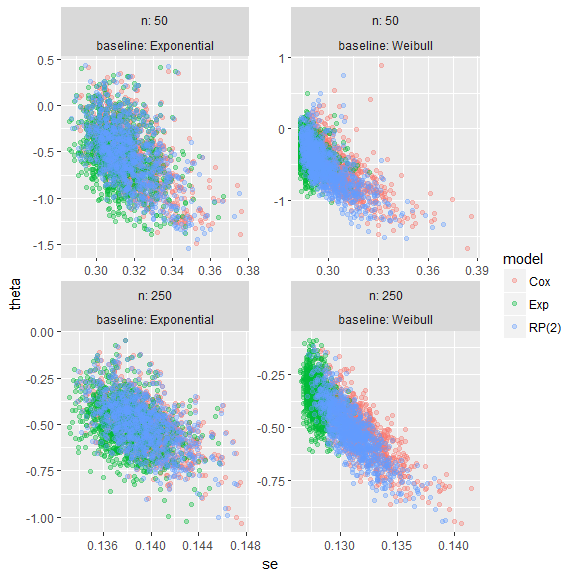Estimates and standard errors mostly overlap, in this settings.

## Lolly plots

Lolly plots are used to present estimates for a given summary statistic with confidence intervals based on Monte Carlo standard errors. They allow to easily compare methods.

Say we are interest in bias:

lolly(s1, sstat = "bias", by = c("n", "baseline"))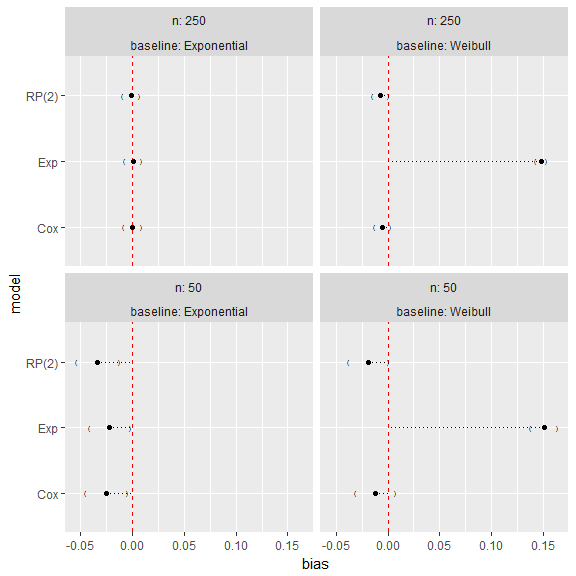It is straightforward to identify the exponential model as yielding biased results when the true baseline hazard is Weibull, irrespectively of the sample size. All the methods also yield biased results with an exponential baseline hazard and a sample size of 50 individuals.

Analogously, for coverage:

lolly(s1, sstat = "cover", by = c("n", "baseline"))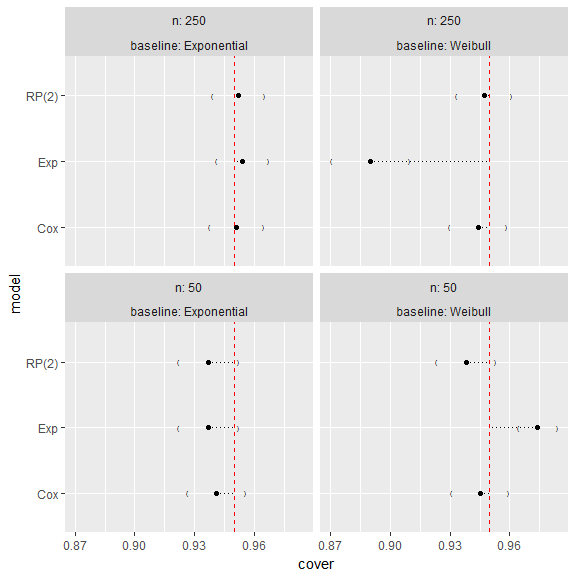## Forest plots and bar plots

Forest plots and bar plots could be an alternative to lolly plots, with similar interpretation:

forest(s1, sstat = "bias", by = c("n", "baseline"))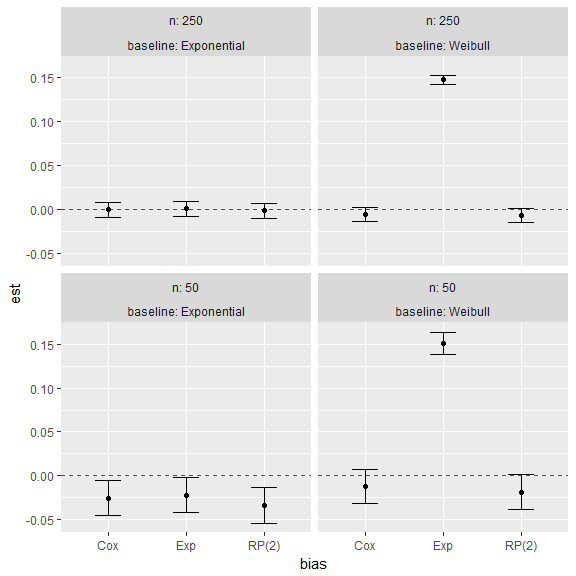forest(s1, sstat = "cover", by = c("n", "baseline"))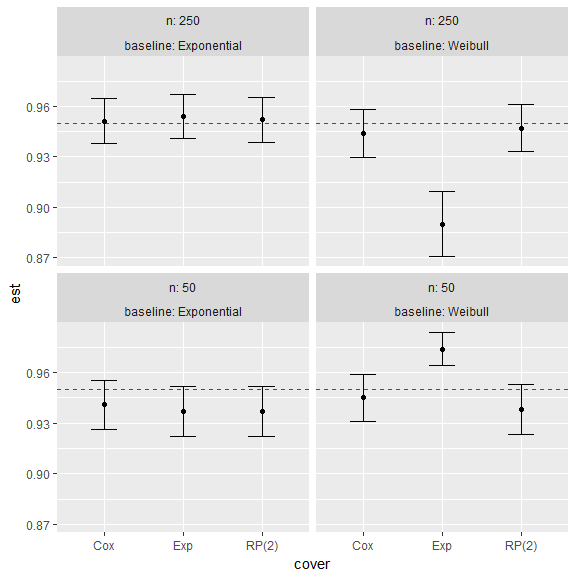bar(s1, sstat = "bias", by = c("n", "baseline"))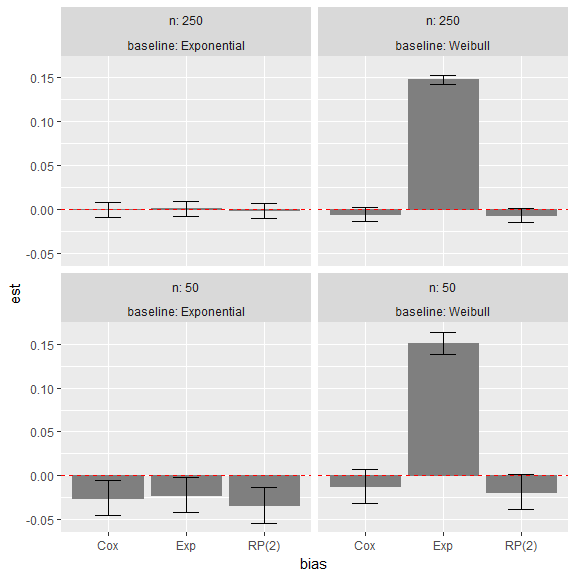bar(s1, sstat = "cover", by = c("n", "baseline"))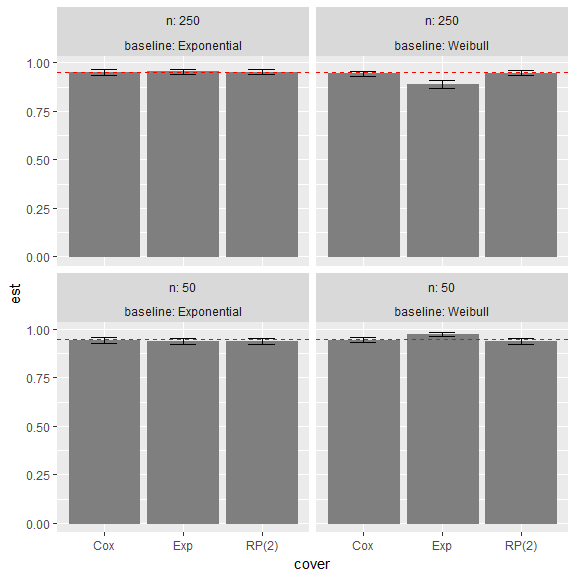## Zip plots

Zip plots, introduced in Morris et al. (2017), help understand coverage by visualising the confidence intervals directly. For each data-generating mechanism and method, the confidence intervals are centile-ranked according to their significance agains the null hypotesis $$H_0: \theta = -0.50$$, assessed via a Wald-type test. This ranking is used for the vertical axis and is plotted against the intervals themselves.

When a method has $$95\%$$ coverage, the colour of the intervals switches at 95 on the vertical axis. Finally, the horizontal lines represent confidence intervals for the estimated coverage based on Monte Carlo standard errors.

zip(s1)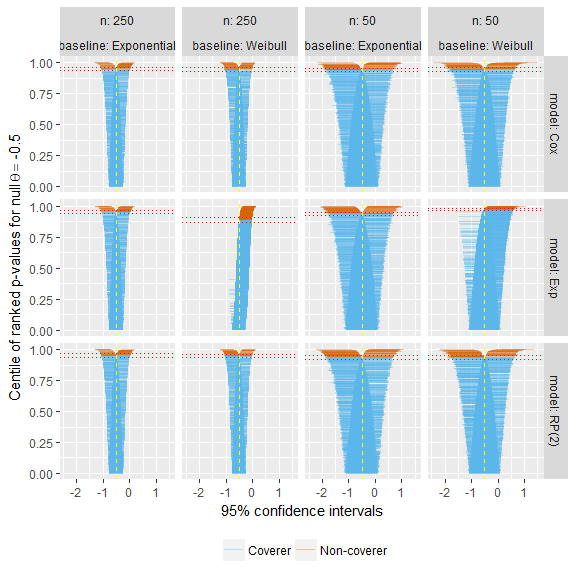The zip plot for the exponential model with $$n = 50$$ and a true Weibull baseline hazard shows that coverage is approximately $$95\%$$; however, there are more intervals to the right of $$\theta = -0.50$$ than to the left: this indicates that the model standard errors must be overestimating the empirical standard error, because coverage is appropriate despite bias.

## Heat plots

Heat plots are a new visualisation that we suggest and include here for the first time. With heat plots, we produce a heat-map-like plot where the filling of each tile represents a given summary statistic, say bias:

heat(s1, sstat = "bias", y = "baseline")
#> Wrapping factors: n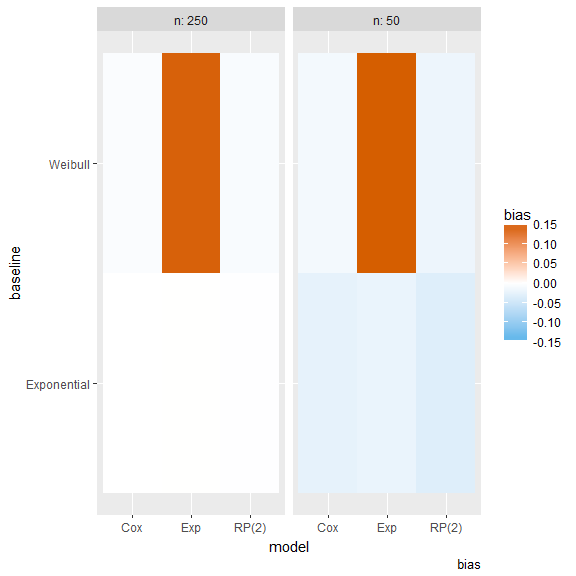heat() requires a factor to be put on the y-axis; by default, the methods are included on the x axis, and all the remaining by-factors will be used for faceting. Therefore, this plot is appropriate when a simulation study includes different methods to be compared and many data-generating mechanisms. Using a heat plot, it is immediate to identify visually which method performs better and under which data-generating mechanisms.

By default, white colour represent the optimal, target value. Using summary statistics with zero as the lower bound (e.g. mean squared error, empirical standard error, etc.), red represents largest (e.g.) worse values. Finally, with summary statistics that can have lower and upper values (e.g. bias), blue colour represents values below the target and red colour represents values above the target. These colors, which are colourblind-safe by default, can be easily changed to taste:

heat(s1, sstat = "bias", y = "baseline", gpars = list(target.colour = "green", low.colour = "yellow", high.colour = "purple"))
#> Wrapping factors: n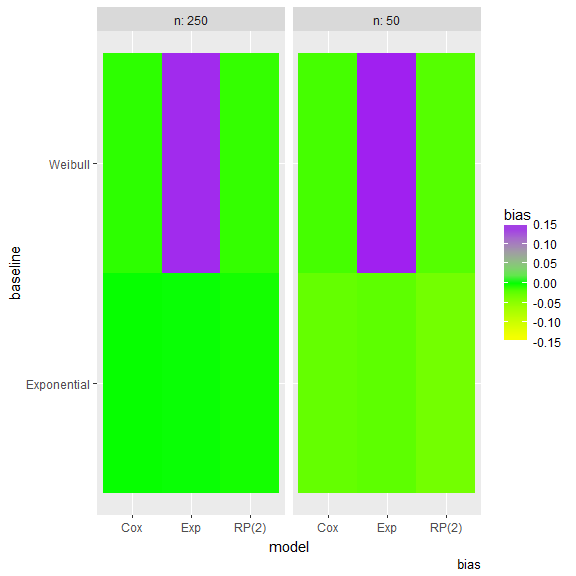It is also possible to add the actual value of each summary statistic and Monte Carlo standard error:

heat(s1, sstat = "bias", y = "baseline", text = TRUE)
#> Wrapping factors: n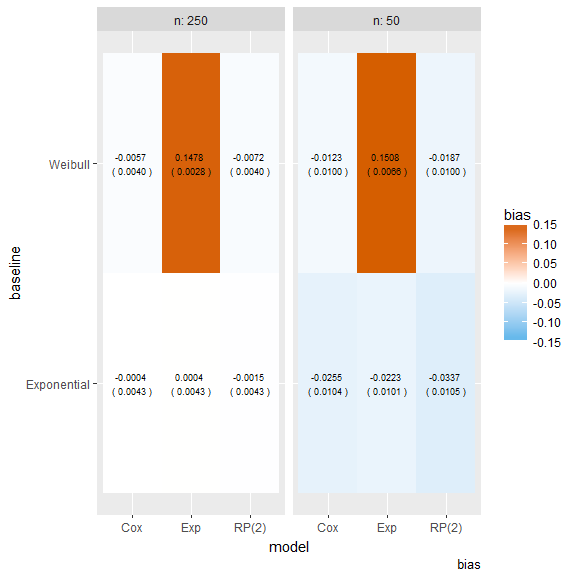# Simulation study with multiple estimands

This second example is using data from a simulation study on misspecification in frailty survival models. This dataset is included in rsimsum and can be loaded with:

data("frailty", package = "rsimsum")

Inspecting the structure of the dataset and the first 15 rows of data:

str(frailty)
#> 'data.frame':    16000 obs. of  6 variables:
#>  $i : int 1 1 1 1 1 1 1 1 1 1 ... #>$ b      : num  0.657 0.84 0.658 0.841 0.639 ...
#>  $se : num 0.126 0.166 0.126 0.18 0.122 ... #>$ par    : chr  "fv" "fv" "fv" "fv" ...
#>  $fv_dist: chr "Gamma" "Gamma" "Gamma" "Gamma" ... #>$ model  : chr  "Cox, Gamma" "Cox, Log-Normal" "RP(P), Gamma" "RP(P), Log-Normal" ...
#>    i          b         se par    fv_dist             model
#> 1  1  0.6569546 0.12569642  fv      Gamma        Cox, Gamma
#> 2  1  0.8396248 0.16633677  fv      Gamma   Cox, Log-Normal
#> 3  1  0.6583130 0.12603540  fv      Gamma      RP(P), Gamma
#> 4  1  0.8410503 0.18048979  fv      Gamma RP(P), Log-Normal
#> 5  1  0.6394722 0.12238081  fv Log-Normal        Cox, Gamma
#> 6  1  0.7573628 0.12350623  fv Log-Normal   Cox, Log-Normal
#> 7  1  0.6405455 0.12269052  fv Log-Normal      RP(P), Gamma
#> 8  1  0.7587269 0.16031007  fv Log-Normal RP(P), Log-Normal
#> 9  1 -0.6059809 0.04951428 trt      Gamma        Cox, Gamma
#> 10 1 -0.6070946 0.04948740 trt      Gamma   Cox, Log-Normal
#> 11 1 -0.6063270 0.04956676 trt      Gamma      RP(P), Gamma
#> 12 1 -0.6073181 0.04957903 trt      Gamma RP(P), Log-Normal
#> 13 1 -0.5193134 0.04807357 trt Log-Normal        Cox, Gamma
#> 14 1 -0.5199779 0.04807639 trt Log-Normal   Cox, Log-Normal
#> 15 1 -0.5193258 0.04809115 trt Log-Normal      RP(P), Gamma

This dataset has two estimands:

unique(frailty[["par"]])
#>  "fv"  "trt"

## Summarise results

We use the simsum function to summarise results:

s2 <- multisimsum(data = frailty, par = "par", true = c(trt = -0.50, fv = 0.75), estvarname = "b", se = "se", methodvar = "model", by = "fv_dist", x = TRUE)
#> ref was not specified, Cox, Gamma set as the reference
s2
#>
#> Call:
#>  multisimsum(data = frailty, par = "par", true = c(trt = -0.5,
#>     fv = 0.75), estvarname = "b", se = "se", methodvar = "model",
#>     by = "fv_dist", x = TRUE)
#>
#> Estimands variable: par
#>  Unique estimands: fv, trt
#>  True values: fv = 0.75, trt = -0.5
#>
#> Method variable: model
#>  Unique methods: Cox, Gamma, Cox, Log-Normal, RP(P), Gamma, RP(P), Log-Normal
#>  Reference method: Cox, Gamma
#>
#> By factors: fv_dist
#>
#> Monte Carlo standard errors were computed.

We call multisimsum with x = TRUE as we want to produce pattern plots and zip plots later on.

summary(s2)
#>
#> Call:
#>  multisimsum(data = frailty, par = "par", true = c(trt = -0.5,
#>     fv = 0.75), estvarname = "b", se = "se", methodvar = "model",
#>     by = "fv_dist", x = TRUE)
#>
#> Estimands variable: par
#>  Unique estimands: fv, trt
#>  True values: fv = 0.75, trt = -0.5
#>
#> Method variable: model
#>  Unique methods: Cox, Gamma, Cox, Log-Normal, RP(P), Gamma, RP(P), Log-Normal
#>  Reference method: Cox, Gamma
#>
#> By factors: fv_dist
#>
#>  ------------------------------------------------------------------------------------------------------------------------------------------------------
#>
#> Summary statistics for estimand 'fv':
#>
#>  Method = Cox, Gamma, fv_dist = Gamma
#>                                                    Estimate   MCSE Lower 95% Upper 95%
#>         Simulations with non-missing estimates/SEs 976.0000     NA        NA        NA
#>                             Average point estimate   0.7376     NA        NA        NA
#>                              Median point estimate   0.7271     NA        NA        NA
#>                             Average standard error   0.0203     NA        NA        NA
#>                              Median standard error   0.0193     NA        NA        NA
#>                             Bias in point estimate  -0.0124 0.0045   -0.0213   -0.0035
#>                           Empirical standard error   0.1421 0.0032    0.1358    0.1484
#>                                 Mean squared error   0.0203 0.0010    0.0184    0.0222
#>  % gain in precision relative to method Cox, Gamma   1.0000 0.0000    1.0000    1.0000
#>                         Model-based standard error   0.1426 0.0008    0.1410    0.1442
#>                 Relative % error in standard error   0.3574 2.3458   -4.2402    4.9550
#>                         Coverage of nominal 95% CI   0.9201 0.0087    0.9031    0.9371
#>          Bias corrected coverage of nominal 95% CI   0.9334 0.0080    0.9178    0.9490
#>                             Power of 5% level test   1.0000 0.0000    1.0000    1.0000
#>
#>  Method = Cox, Log-Normal, fv_dist = Gamma
#>                                                     Estimate   MCSE Lower 95% Upper 95%
#>         Simulations with non-missing estimates/SEs 1000.0000     NA        NA        NA
#>                             Average point estimate    0.9799     NA        NA        NA
#>                              Median point estimate    0.9566     NA        NA        NA
#>                             Average standard error    0.0600     NA        NA        NA
#>                              Median standard error    0.0483     NA        NA        NA
#>                             Bias in point estimate    0.2299 0.0076    0.2150    0.2448
#>                           Empirical standard error    0.2406 0.0054    0.2301    0.2512
#>                                 Mean squared error    0.1107 0.0055    0.1000    0.1214
#>  % gain in precision relative to method Cox, Gamma    0.3487 0.0061    0.3367    0.3608
#>                         Model-based standard error    0.2449 0.0027    0.2396    0.2503
#>                 Relative % error in standard error    1.7896 2.5444   -3.1973    6.7765
#>                         Coverage of nominal 95% CI    0.9220 0.0085    0.9054    0.9386
#>          Bias corrected coverage of nominal 95% CI    0.8980 0.0096    0.8792    0.9168
#>                             Power of 5% level test    1.0000 0.0000    1.0000    1.0000
#>
#>  Method = RP(P), Gamma, fv_dist = Gamma
#>                                                    Estimate   MCSE Lower 95% Upper 95%
#>         Simulations with non-missing estimates/SEs 971.0000     NA        NA        NA
#>                             Average point estimate   0.7321     NA        NA        NA
#>                              Median point estimate   0.7225     NA        NA        NA
#>                             Average standard error   0.0202     NA        NA        NA
#>                              Median standard error   0.0191     NA        NA        NA
#>                             Bias in point estimate  -0.0179 0.0044   -0.0266   -0.0092
#>                           Empirical standard error   0.1387 0.0031    0.1325    0.1448
#>                                 Mean squared error   0.0195 0.0009    0.0178    0.0213
#>  % gain in precision relative to method Cox, Gamma   1.0500 0.0005    1.0491    1.0510
#>                         Model-based standard error   0.1420 0.0008    0.1404    0.1435
#>                 Relative % error in standard error   2.3922 2.3945   -2.3009    7.0852
#>                         Coverage of nominal 95% CI   0.9300 0.0082    0.9139    0.9460
#>          Bias corrected coverage of nominal 95% CI   0.9434 0.0074    0.9288    0.9579
#>                             Power of 5% level test   1.0000 0.0000    1.0000    1.0000
#>
#>  Method = RP(P), Log-Normal, fv_dist = Gamma
#>                                                     Estimate   MCSE Lower 95% Upper 95%
#>         Simulations with non-missing estimates/SEs 1000.0000     NA        NA        NA
#>                             Average point estimate    0.9847     NA        NA        NA
#>                              Median point estimate    0.9597     NA        NA        NA
#>                             Average standard error    0.0498     NA        NA        NA
#>                              Median standard error    0.0442     NA        NA        NA
#>                             Bias in point estimate    0.2347 0.0077    0.2196    0.2499
#>                           Empirical standard error    0.2438 0.0055    0.2331    0.2545
#>                                 Mean squared error    0.1145 0.0057    0.1033    0.1256
#>  % gain in precision relative to method Cox, Gamma    0.3397 0.0059    0.3281    0.3512
#>                         Model-based standard error    0.2232 0.0019    0.2195    0.2269
#>                 Relative % error in standard error   -8.4531 2.1885  -12.7425   -4.1636
#>                         Coverage of nominal 95% CI    0.9030 0.0094    0.8847    0.9213
#>          Bias corrected coverage of nominal 95% CI    0.8930 0.0098    0.8738    0.9122
#>                             Power of 5% level test    1.0000 0.0000    1.0000    1.0000
#>
#>  Method = Cox, Gamma, fv_dist = Log-Normal
#>                                                    Estimate   MCSE Lower 95% Upper 95%
#>         Simulations with non-missing estimates/SEs 957.0000     NA        NA        NA
#>                             Average point estimate   0.6436     NA        NA        NA
#>                              Median point estimate   0.6365     NA        NA        NA
#>                             Average standard error   0.0156     NA        NA        NA
#>                              Median standard error   0.0149     NA        NA        NA
#>                             Bias in point estimate  -0.1064 0.0043   -0.1148   -0.0980
#>                           Empirical standard error   0.1320 0.0030    0.1261    0.1379
#>                                 Mean squared error   0.0287 0.0010    0.0267    0.0308
#>  % gain in precision relative to method Cox, Gamma   1.0000 0.0000    1.0000    1.0000
#>                         Model-based standard error   0.1249 0.0008    0.1233    0.1264
#>                 Relative % error in standard error  -5.3912 2.2447   -9.7907   -0.9918
#>                         Coverage of nominal 95% CI   0.7503 0.0140    0.7228    0.7777
#>          Bias corrected coverage of nominal 95% CI   0.9164 0.0089    0.8989    0.9339
#>                             Power of 5% level test   1.0000 0.0000    1.0000    1.0000
#>
#>  Method = Cox, Log-Normal, fv_dist = Log-Normal
#>                                                     Estimate   MCSE Lower 95% Upper 95%
#>         Simulations with non-missing estimates/SEs 1000.0000     NA        NA        NA
#>                             Average point estimate    0.7325     NA        NA        NA
#>                              Median point estimate    0.7182     NA        NA        NA
#>                             Average standard error    0.0230     NA        NA        NA
#>                              Median standard error    0.0206     NA        NA        NA
#>                             Bias in point estimate   -0.0175 0.0049   -0.0271   -0.0079
#>                           Empirical standard error    0.1554 0.0035    0.1485    0.1622
#>                                 Mean squared error    0.0244 0.0011    0.0222    0.0266
#>  % gain in precision relative to method Cox, Gamma    0.7217 0.0150    0.6922    0.7512
#>                         Model-based standard error    0.1517 0.0013    0.1493    0.1542
#>                 Relative % error in standard error   -2.3382 2.3296   -6.9040    2.2276
#>                         Coverage of nominal 95% CI    0.9020 0.0094    0.8836    0.9204
#>          Bias corrected coverage of nominal 95% CI    0.9130 0.0089    0.8955    0.9305
#>                             Power of 5% level test    1.0000 0.0000    1.0000    1.0000
#>
#>  Method = RP(P), Gamma, fv_dist = Log-Normal
#>                                                    Estimate   MCSE Lower 95% Upper 95%
#>         Simulations with non-missing estimates/SEs 997.0000     NA        NA        NA
#>                             Average point estimate   0.6434     NA        NA        NA
#>                              Median point estimate   0.6324     NA        NA        NA
#>                             Average standard error   0.0158     NA        NA        NA
#>                              Median standard error   0.0149     NA        NA        NA
#>                             Bias in point estimate  -0.1066 0.0041   -0.1147   -0.0985
#>                           Empirical standard error   0.1307 0.0029    0.1249    0.1364
#>                                 Mean squared error   0.0284 0.0010    0.0264    0.0304
#>  % gain in precision relative to method Cox, Gamma   1.0205 0.0005    1.0196    1.0214
#>                         Model-based standard error   0.1258 0.0007    0.1244    0.1272
#>                 Relative % error in standard error  -3.7112 2.2295   -8.0809    0.6586
#>                         Coverage of nominal 95% CI   0.7683 0.0134    0.7421    0.7945
#>          Bias corrected coverage of nominal 95% CI   0.9308 0.0080    0.9150    0.9465
#>                             Power of 5% level test   1.0000 0.0000    1.0000    1.0000
#>
#>  Method = RP(P), Log-Normal, fv_dist = Log-Normal
#>                                                     Estimate   MCSE Lower 95% Upper 95%
#>         Simulations with non-missing estimates/SEs 1000.0000     NA        NA        NA
#>                             Average point estimate    0.7348     NA        NA        NA
#>                              Median point estimate    0.7199     NA        NA        NA
#>                             Average standard error    0.0254     NA        NA        NA
#>                              Median standard error    0.0235     NA        NA        NA
#>                             Bias in point estimate   -0.0152 0.0050   -0.0250   -0.0055
#>                           Empirical standard error    0.1570 0.0035    0.1501    0.1639
#>                                 Mean squared error    0.0248 0.0012    0.0226    0.0271
#>  % gain in precision relative to method Cox, Gamma    0.7069 0.0146    0.6783    0.7355
#>                         Model-based standard error    0.1594 0.0011    0.1573    0.1615
#>                 Relative % error in standard error    1.5422 2.3708   -3.1044    6.1888
#>                         Coverage of nominal 95% CI    0.9280 0.0082    0.9120    0.9440
#>          Bias corrected coverage of nominal 95% CI    0.9360 0.0077    0.9208    0.9512
#>                             Power of 5% level test    1.0000 0.0000    1.0000    1.0000
#>
#>  ------------------------------------------------------------------------------------------------------------------------------------------------------
#>
#> Summary statistics for estimand 'trt':
#>
#>  Method = Cox, Gamma, fv_dist = Gamma
#>                                                     Estimate   MCSE Lower 95% Upper 95%
#>         Simulations with non-missing estimates/SEs 1000.0000     NA        NA        NA
#>                             Average point estimate   -0.5006     NA        NA        NA
#>                              Median point estimate   -0.5011     NA        NA        NA
#>                             Average standard error    0.0026     NA        NA        NA
#>                              Median standard error    0.0026     NA        NA        NA
#>                             Bias in point estimate   -0.0006 0.0016   -0.0037    0.0026
#>                           Empirical standard error    0.0508 0.0011    0.0485    0.0530
#>                                 Mean squared error    0.0026 0.0001    0.0023    0.0028
#>  % gain in precision relative to method Cox, Gamma    1.0000 0.0000    1.0000    1.0000
#>                         Model-based standard error    0.0506 0.0000    0.0506    0.0507
#>                 Relative % error in standard error   -0.2017 2.2340   -4.5803    4.1769
#>                         Coverage of nominal 95% CI    0.9500 0.0069    0.9365    0.9635
#>          Bias corrected coverage of nominal 95% CI    0.9500 0.0069    0.9365    0.9635
#>                             Power of 5% level test    1.0000 0.0000    1.0000    1.0000
#>
#>  Method = Cox, Log-Normal, fv_dist = Gamma
#>                                                     Estimate   MCSE Lower 95% Upper 95%
#>         Simulations with non-missing estimates/SEs 1000.0000     NA        NA        NA
#>                             Average point estimate   -0.5013     NA        NA        NA
#>                              Median point estimate   -0.5021     NA        NA        NA
#>                             Average standard error    0.0026     NA        NA        NA
#>                              Median standard error    0.0026     NA        NA        NA
#>                             Bias in point estimate   -0.0013 0.0016   -0.0045    0.0018
#>                           Empirical standard error    0.0509 0.0011    0.0486    0.0531
#>                                 Mean squared error    0.0026 0.0001    0.0023    0.0028
#>  % gain in precision relative to method Cox, Gamma    0.9955 0.0011    0.9933    0.9978
#>                         Model-based standard error    0.0507 0.0000    0.0506    0.0508
#>                 Relative % error in standard error   -0.3890 2.2298   -4.7594    3.9813
#>                         Coverage of nominal 95% CI    0.9490 0.0070    0.9354    0.9626
#>          Bias corrected coverage of nominal 95% CI    0.9500 0.0069    0.9365    0.9635
#>                             Power of 5% level test    1.0000 0.0000    1.0000    1.0000
#>
#>  Method = RP(P), Gamma, fv_dist = Gamma
#>                                                    Estimate   MCSE Lower 95% Upper 95%
#>         Simulations with non-missing estimates/SEs 971.0000     NA        NA        NA
#>                             Average point estimate  -0.5003     NA        NA        NA
#>                              Median point estimate  -0.5010     NA        NA        NA
#>                             Average standard error   0.0026     NA        NA        NA
#>                              Median standard error   0.0026     NA        NA        NA
#>                             Bias in point estimate  -0.0003 0.0016   -0.0035    0.0029
#>                           Empirical standard error   0.0506 0.0011    0.0484    0.0529
#>                                 Mean squared error   0.0026 0.0001    0.0023    0.0028
#>  % gain in precision relative to method Cox, Gamma   1.0044 0.0008    1.0027    1.0061
#>                         Model-based standard error   0.0506 0.0000    0.0505    0.0507
#>                 Relative % error in standard error  -0.0544 2.2704   -4.5043    4.3955
#>                         Coverage of nominal 95% CI   0.9506 0.0070    0.9369    0.9642
#>          Bias corrected coverage of nominal 95% CI   0.9506 0.0070    0.9369    0.9642
#>                             Power of 5% level test   1.0000 0.0000    1.0000    1.0000
#>
#>  Method = RP(P), Log-Normal, fv_dist = Gamma
#>                                                     Estimate   MCSE Lower 95% Upper 95%
#>         Simulations with non-missing estimates/SEs 1000.0000     NA        NA        NA
#>                             Average point estimate   -0.5015     NA        NA        NA
#>                              Median point estimate   -0.5025     NA        NA        NA
#>                             Average standard error    0.0026     NA        NA        NA
#>                              Median standard error    0.0026     NA        NA        NA
#>                             Bias in point estimate   -0.0015 0.0016   -0.0047    0.0016
#>                           Empirical standard error    0.0509 0.0011    0.0487    0.0531
#>                                 Mean squared error    0.0026 0.0001    0.0023    0.0028
#>  % gain in precision relative to method Cox, Gamma    0.9942 0.0016    0.9910    0.9974
#>                         Model-based standard error    0.0507 0.0000    0.0506    0.0508
#>                 Relative % error in standard error   -0.4330 2.2288   -4.8014    3.9355
#>                         Coverage of nominal 95% CI    0.9500 0.0069    0.9365    0.9635
#>          Bias corrected coverage of nominal 95% CI    0.9490 0.0070    0.9354    0.9626
#>                             Power of 5% level test    1.0000 0.0000    1.0000    1.0000
#>
#>  Method = Cox, Gamma, fv_dist = Log-Normal
#>                                                     Estimate   MCSE Lower 95% Upper 95%
#>         Simulations with non-missing estimates/SEs 1000.0000     NA        NA        NA
#>                             Average point estimate   -0.5006     NA        NA        NA
#>                              Median point estimate   -0.5014     NA        NA        NA
#>                             Average standard error    0.0023     NA        NA        NA
#>                              Median standard error    0.0022     NA        NA        NA
#>                             Bias in point estimate   -0.0006 0.0015   -0.0035    0.0024
#>                           Empirical standard error    0.0474 0.0011    0.0453    0.0494
#>                                 Mean squared error    0.0022 0.0001    0.0020    0.0024
#>  % gain in precision relative to method Cox, Gamma    1.0000 0.0000    1.0000    1.0000
#>                         Model-based standard error    0.0475 0.0000    0.0474    0.0475
#>                 Relative % error in standard error    0.2507 2.2433   -4.1461    4.6474
#>                         Coverage of nominal 95% CI    0.9410 0.0075    0.9264    0.9556
#>          Bias corrected coverage of nominal 95% CI    0.9420 0.0074    0.9275    0.9565
#>                             Power of 5% level test    1.0000 0.0000    1.0000    1.0000
#>
#>  Method = Cox, Log-Normal, fv_dist = Log-Normal
#>                                                     Estimate   MCSE Lower 95% Upper 95%
#>         Simulations with non-missing estimates/SEs 1000.0000     NA        NA        NA
#>                             Average point estimate   -0.5014     NA        NA        NA
#>                              Median point estimate   -0.5021     NA        NA        NA
#>                             Average standard error    0.0023     NA        NA        NA
#>                              Median standard error    0.0022     NA        NA        NA
#>                             Bias in point estimate   -0.0014 0.0015   -0.0044    0.0015
#>                           Empirical standard error    0.0474 0.0011    0.0453    0.0495
#>                                 Mean squared error    0.0022 0.0001    0.0020    0.0024
#>  % gain in precision relative to method Cox, Gamma    0.9986 0.0014    0.9959    1.0013
#>                         Model-based standard error    0.0475 0.0000    0.0474    0.0475
#>                 Relative % error in standard error    0.1815 2.2417   -4.2123    4.5752
#>                         Coverage of nominal 95% CI    0.9420 0.0074    0.9275    0.9565
#>          Bias corrected coverage of nominal 95% CI    0.9400 0.0075    0.9253    0.9547
#>                             Power of 5% level test    1.0000 0.0000    1.0000    1.0000
#>
#>  Method = RP(P), Gamma, fv_dist = Log-Normal
#>                                                    Estimate   MCSE Lower 95% Upper 95%
#>         Simulations with non-missing estimates/SEs 997.0000     NA        NA        NA
#>                             Average point estimate  -0.5006     NA        NA        NA
#>                              Median point estimate  -0.5014     NA        NA        NA
#>                             Average standard error   0.0023     NA        NA        NA
#>                              Median standard error   0.0022     NA        NA        NA
#>                             Bias in point estimate  -0.0006 0.0015   -0.0036    0.0023
#>                           Empirical standard error   0.0473 0.0011    0.0453    0.0494
#>                                 Mean squared error   0.0022 0.0001    0.0020    0.0024
#>  % gain in precision relative to method Cox, Gamma   1.0009 0.0009    0.9992    1.0026
#>                         Model-based standard error   0.0475 0.0000    0.0474    0.0476
#>                 Relative % error in standard error   0.3319 2.2485   -4.0750    4.7389
#>                         Coverage of nominal 95% CI   0.9428 0.0074    0.9284    0.9572
#>          Bias corrected coverage of nominal 95% CI   0.9428 0.0074    0.9284    0.9572
#>                             Power of 5% level test   1.0000 0.0000    1.0000    1.0000
#>
#>  Method = RP(P), Log-Normal, fv_dist = Log-Normal
#>                                                     Estimate   MCSE Lower 95% Upper 95%
#>         Simulations with non-missing estimates/SEs 1000.0000     NA        NA        NA
#>                             Average point estimate   -0.5016     NA        NA        NA
#>                              Median point estimate   -0.5022     NA        NA        NA
#>                             Average standard error    0.0023     NA        NA        NA
#>                              Median standard error    0.0022     NA        NA        NA
#>                             Bias in point estimate   -0.0016 0.0015   -0.0045    0.0013
#>                           Empirical standard error    0.0474 0.0011    0.0453    0.0495
#>                                 Mean squared error    0.0022 0.0001    0.0020    0.0024
#>  % gain in precision relative to method Cox, Gamma    0.9979 0.0016    0.9948    1.0010
#>                         Model-based standard error    0.0475 0.0000    0.0474    0.0476
#>                 Relative % error in standard error    0.2101 2.2424   -4.1849    4.6051
#>                         Coverage of nominal 95% CI    0.9430 0.0073    0.9286    0.9574
#>          Bias corrected coverage of nominal 95% CI    0.9410 0.0075    0.9264    0.9556
#>                             Power of 5% level test    1.0000 0.0000    1.0000    1.0000

## Patterns

Plotting pattern plots for a given estimand:

pattern(s2, par = "trt")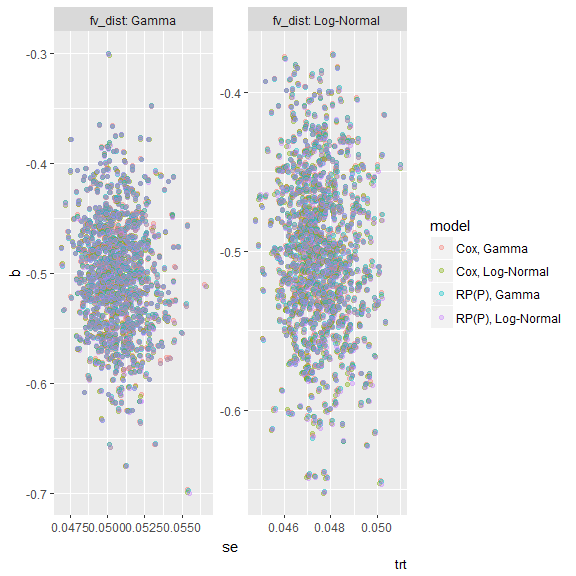Alternatively, plotting pattern plots for all estimands:

pattern(s2)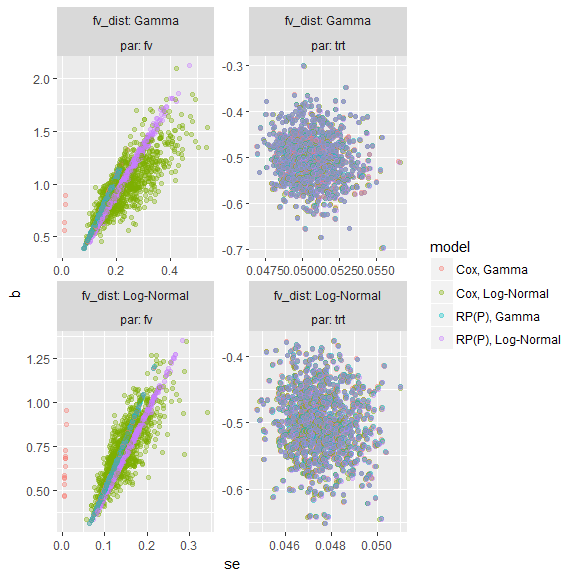Estimates and standard errors mostly overlap, in this settings.

## Lolly plots

Lolly plot for bias of treatment effect:

lolly(s2, sstat = "bias", par = "trt", by = "fv_dist")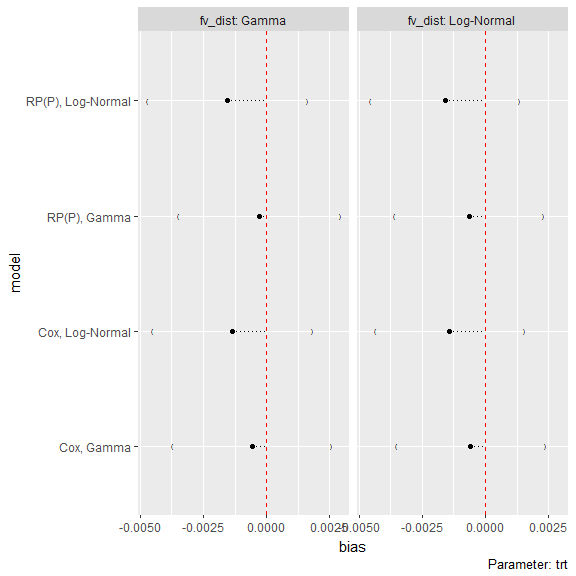Lolly plot for coverage of frailty variance:

lolly(s2, sstat = "cover", par = "fv", by = "fv_dist")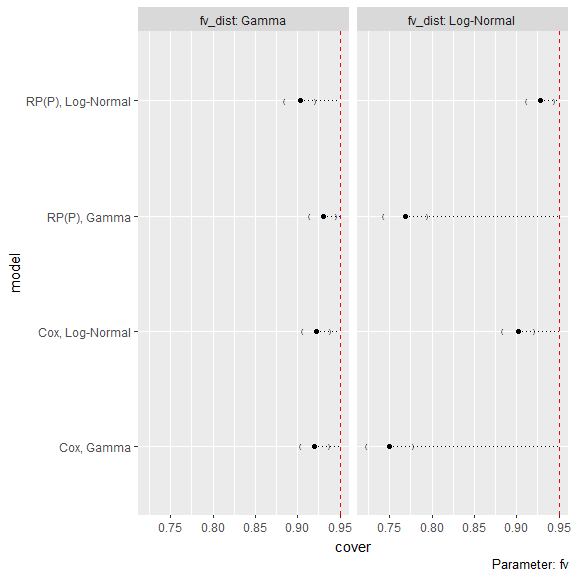It is also possible to not select a single estimand and plot them both, say for bias:

lolly(s2, sstat = "bias", by = "fv_dist")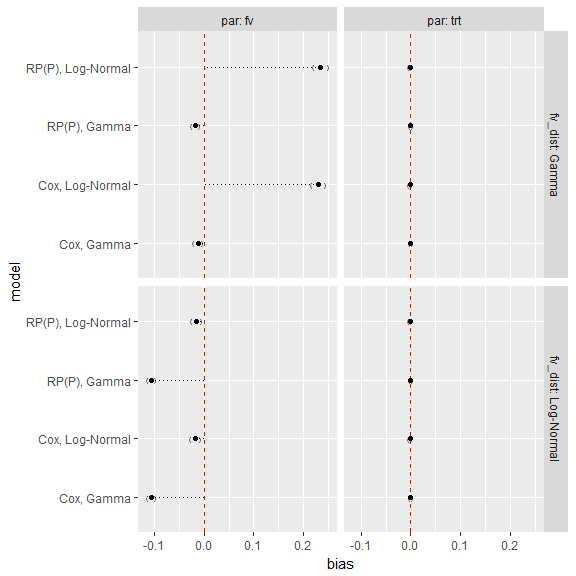## Forest plots and bar plots

Forest plots for bias and coverage of treatment effect:

forest(s2, sstat = "bias", par = "trt", by = "fv_dist")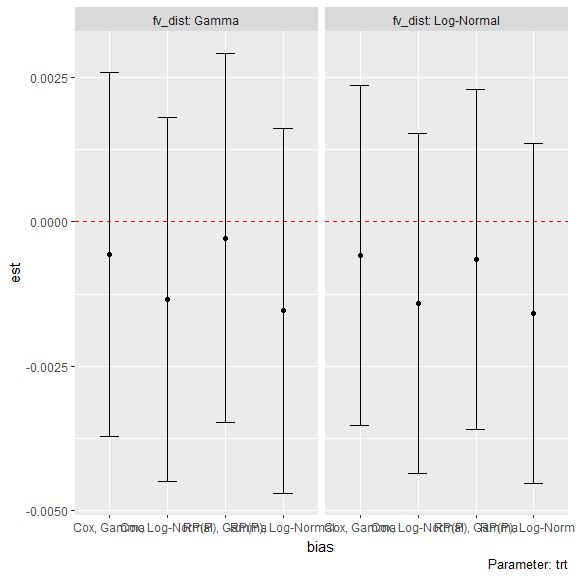forest(s2, sstat = "cover", par = "fv", by = "fv_dist")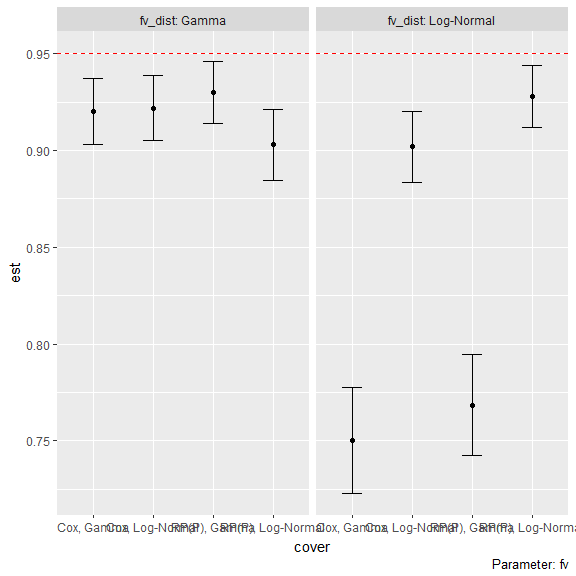Bar plots for bias and coverage of treatment effect:

bar(s2, sstat = "bias", par = "trt", by = "fv_dist")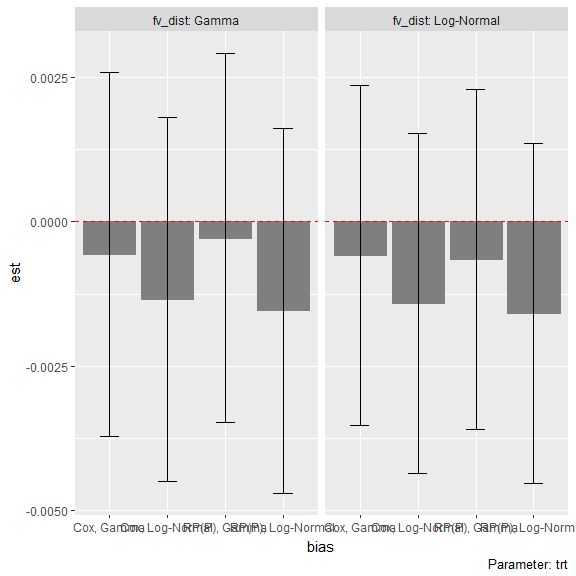bar(s2, sstat = "cover", par = "fv", by = "fv_dist")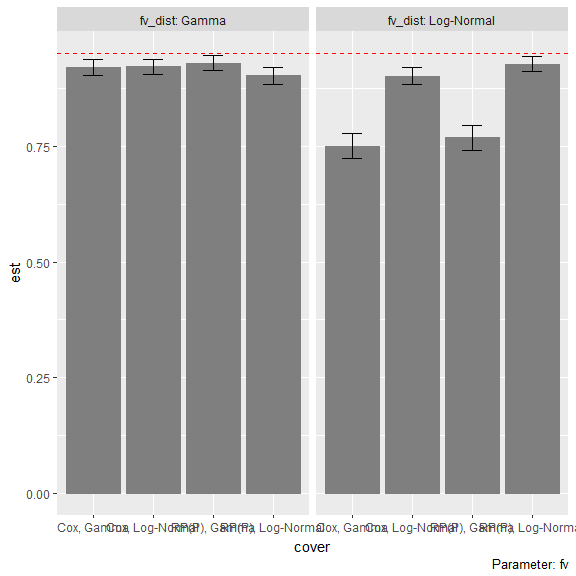## Zip plots

Say we want to further investigate coverage of the frailty variance after seeing the lolly plot:

zip(s2, par = "fv")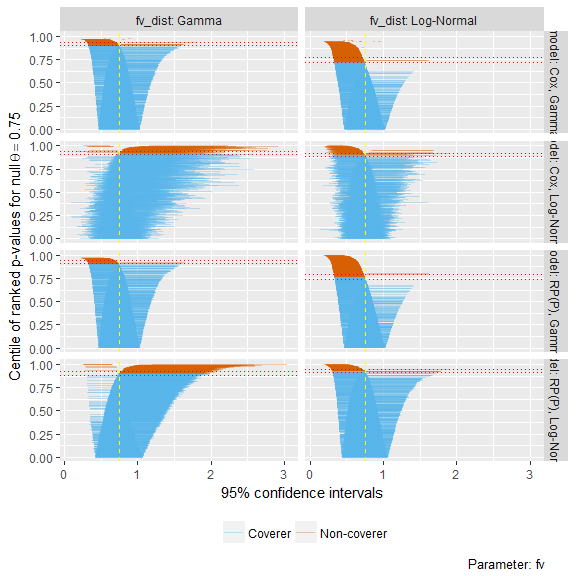## Heat plots

Finally, we also plot coverage of the frailty variance using a heat plot:

heat(s2, par = "fv", sstat = "cover", y = "fv_dist")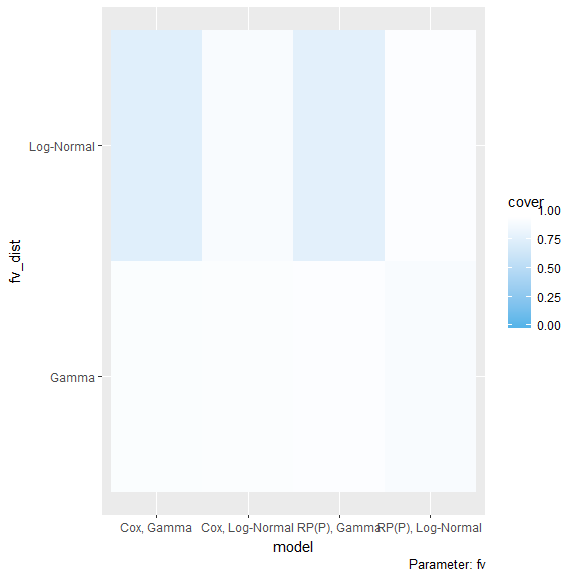heat(s2, par = "fv", sstat = "cover", y = "fv_dist", text = TRUE)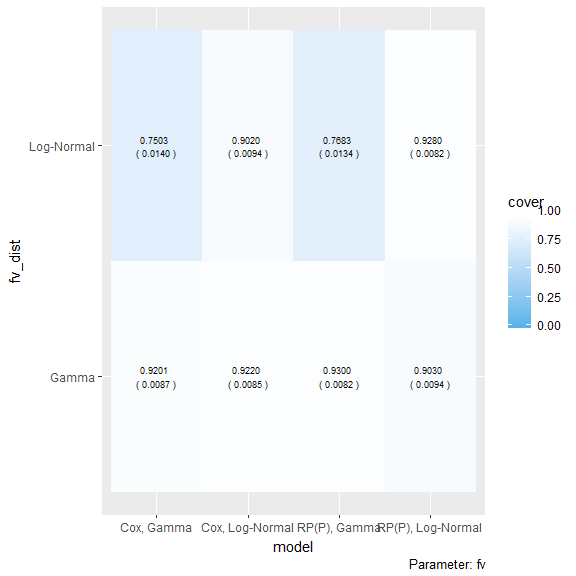# Combining plots with additional geom_*, theme_*, etc.

Each method introduced with this vignette returns ggplot objects that can be easily combined with any other geom_*, theme_*, scale_*.

For instance:

1. Adding a method-specific smoother to each panel of a pattern plot, to better visualise trends:
pattern(s1) +
geom_smooth()
#> geom_smooth() using method = 'gam'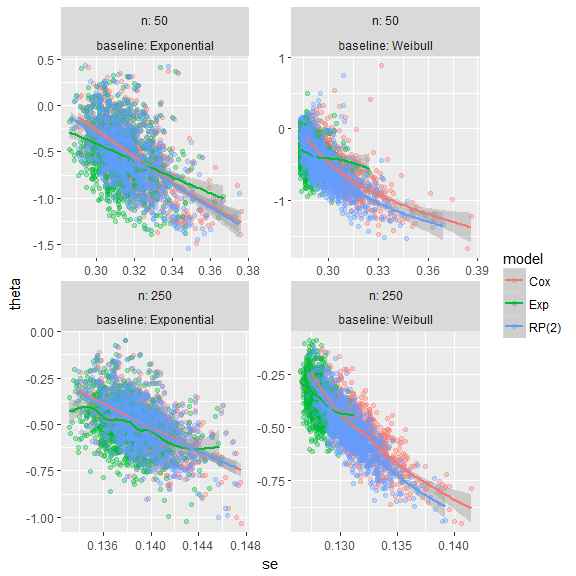heat(s1, sstat = "bias", y = "baseline") +
#> Wrapping factors: n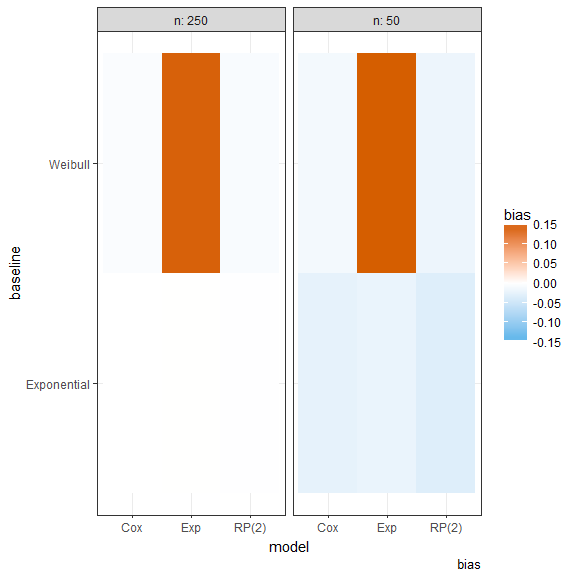heat(s1, sstat = "bias", y = "baseline") +
#> Scale for 'fill' is already present. Adding another scale for 'fill', which will replace the existing scale.